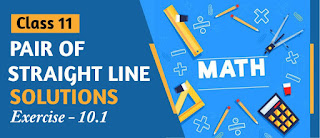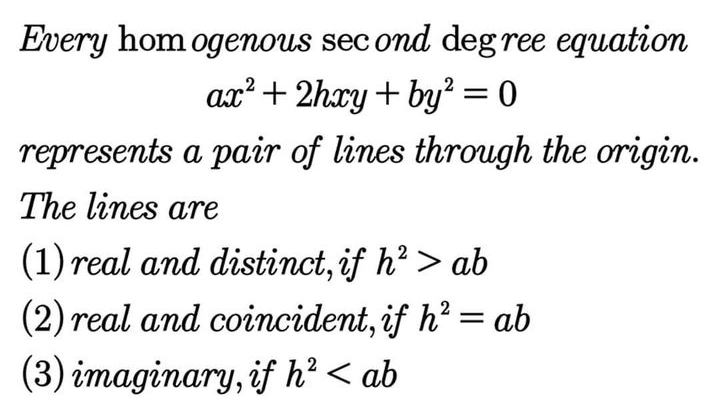# Pair of Straight Line Class 11 Mathematics Solutions | Exercise - 10.1## Chapter - 10  Pair of Straight Line

We all have to study Pair of Straight Line chapter in class 11 mathematics. Though we study about this chapter, many of us don't know what exactly we study in this chapter. So let me tell you, what subtopics we need to study, in this chapter.

Basically we study about general equation of second dregree and homogenous equation in this chapter. So let's have a quick knowledge about these subtopucs before we proceed to the solutions section.

### General Equation of Second Degree

Let A₁x + B₁y + C₁= 0 ------------------ (i)
and A₂x + B₂y + C₂ = 0 ------------------- (ii)
be the equations of two straight lines.

Consider the equation,
(A₁x + B₁y + C₁) (A₂x + B₂y + C₂) = 0 ------------------ (iii)

The coordinates of any point lying in straight line (1) will satisfy (3) also. Hence all points on the straight line (1) lie on (3). Similarly all points lying on the straight line (2) lie on (3).

Conversely, the coordinates of any point lying on (3) will satisfy (1) or (2) or both. Hence equation (3) represents a pair of the straight lines given by (1) and (2). If the left hand side of equation (3) be expanded we get an equation of second degree in x and y, i.e. an equation of the form,
ax² + 2hxy + by + 2gx + 2f + c=0

Thus we see that the equation of a pair of lines is a second degree equation. But the converse is not always true. As we shall see later, equations of second degree will represent a pair of straight lines only if the left hand side can be resolved into two linear factors.

Homogeneous Equation

An equation with two variables x and y in which each term has the same degree is known as a homogeneous equation. If the degree of each term is 2, then the equation is known as the homogeneous equation of degree two or a second degree homogeneous equation. The most general form of a homogeneous equation of degree two is ax² + 2hxy + by² = 0.

Homogeneous Equation of Second Degree

The Homogeneous Equation of Second Degree ax² + 2hxy + by² = 0, always Represents a Pair of Straight Lines through the Origin.

The homogeneous equation of second degree in x and y is ax² + 2hxy + by² = 0.
The equation can be written as:
y² + (2h/b)xy + ax²/b = 0,   if b ≠ 0### Exercise - 10.1

This PDF has all the solutions of exercise 10.1. Now, this chapter has only one exercise and it is named as exercise 10.1. So don't go on searching for exercise10.2.

NoteScroll the PDF to view all Solution

You are not allowed to post this PDF in any website or social platform without permission.

## How can I score good marks in Mathematics?

If you are asking me how to score good marks in mathematics then I may not be able to help you 100%. It is because your marks depends on your hardwork. The more you practise, the more you score marks in your exam.

Now to be honest, I am also very weak in mathematics. I practice a lot but also secure very less marks. Despite that I keep on trying and working hard. It is probably because someday my hardworking will certainly get paid.

So if you are also feeling low about not being able to score good marks then you shouldn't lose your hope. Keep on practicing everyday. You have chosen mathematics in grade 11 and it was your own choice. You shouldn't regret on your choice.

## How do I avoid silly mistakes in math?

Mathematics can be a challenging subject, and making silly mistakes can often lead to incorrect answers. However, there are effective strategies that can help you minimize these errors and improve your math skills. In this article, we will explore three practical approaches to avoiding silly mistakes in math.

1. Take your time: Don't rush through any problems. Try to read each question carefully and break down complex problems into smaller steps. Make sure you have understood each step before moving forward.

2. Practice regularly: Practice is very much essential for improving your math skills. Work through a variety of problems using different resources. Keep on practicing regularly as it helps you to recognize patterns, and reduces mistakes.

Disclaimer: This website is made for educational purposes. If you find any content that belongs to you then contact us through the contact form. We will remove that content from our as soon as possible.

If you have any queries then feel free to comment down.

No Comment